# Compound Probability

In this lesson you will learn about compound events, composed of independent and dependent events. You will see how to create probability models that show the sample space for compound events and how to calculate arrangements by using factorials. You will also learn how to set up simulations for experiments and determine outcomes from these simulations.

Think about compound sentences. They are composed of at least two complete sentences. Well, a compound event is composed of two or more separate events. In order to find the probability of a compound event, it is important to understand if it is an independent or dependent event.

Independent events are events in which the outcome of one event does not affect the probability of the other.

• Example: Toss a coin twice – The result of one toss has no effect on the result of the other toss.
• Example: Draw a marble from a bag of assorted marbles, replace the marble, and draw another.

Dependent events are events in which the outcome of one event does affect the probability of the other.

• Example: Draw a marble from a bag of assorted marbles, and then draw another marble from the bag without replacing the first marble.
• Example: One student selects a book from the class library, and then another student selects a book from the remaining books.

The probability of a compound event is the ratio of favorable outcomes to total outcomes in the sample space for which the compound events happen. Remember that you can use sample spaces (tree diagrams, tables, and organized lists) to find the probability of compound events. You may also use the rules below:

• Probability of independent events (Multiply the probabilities): P (A and B) = P (A) * P (B)
• Probability of dependent events: (Calculate the probability of the first event. Calculate the probability that the second event would occur if the first event had already occurred.) In other words, multiply the probability of the first by the probability of the second AFTER the first: P(A and B) = P(A) x P(B after A)

### Example

Kelly and her friends are playing a board game. To move her game piece, she must roll the same number on two number cubes (1-6). Represent the sample space and find all of the ways that Kelly could roll the same number.

You can create a Tree Diagram to show the sample space: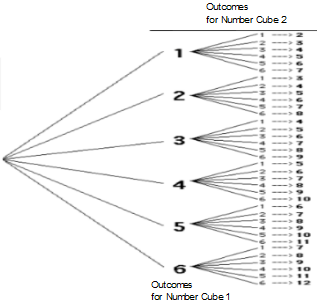Notice that there are 36 outcomes in the sample space and that there are 6 outcomes where the number cubes are the same. Kelly could roll the same number 1 out of 6 times.

You can make a table to show the sample space:

 1, 1 1, 2 1, 3 1, 4 1, 5 1, 6 2, 1 2, 2 2, 3 2, 4 2, 5 2, 6 3, 1 3, 2 3, 3 3, 4 3, 5 3, 6 4, 1 4, 2 4, 3 4, 4 4, 5 4, 6 5, 1 5, 2 5, 3 5, 4 5, 5 5, 6 6, 1 6, 2 6, 3 6, 4 6, 5 6, 6

The sample space shows there are 36 outcomes and that 6 of those are doubles.

You can also use the formula for probability of independent events to find the probability. Remember that rolling two number cubes represents two independent events.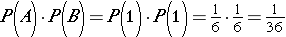. The probability of rolling doubles is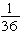. There are 6 numbers on each cube so you add the probabilities together to see the probability of getting the same number.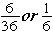### Example

The letters in the word mathematics are placed in a container.

If two letters are chosen at random, what is the probability that both will be consonants?

Since the first letter drawn is not replaced, the sample space is different for the second letter, so the events are dependent. Find the probability that the first letter selected is a consonant.

The sample space has 11 outcomes. 7 of those are consonants.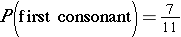If the first letter selected was a consonant, which changes the number of letters in the container to 10 and the total number of consonants to 6. Find the probability that the second letter selected is a consonant.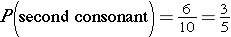Now use the formula to find the probability of choosing two consonants.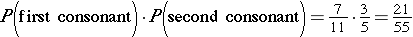The probability of selecting two letters that are both consonants is.

Before using a strategy to find the probability of compound events, determine whether the event is dependent or independent.

Can you tell whether the following examples are independent or dependent?

1. Drawing a green ball from a bucket and then drawing a yellow ball without replacing the first.2. Spinning a 4 on a spinner three times in a row.
3. Flipping a coin and getting heads-up, then flipping the same coin and getting tails-up.
4. Selecting a two green marbles from a bag of assorted color marbles.(Check answers)

## Compound Probability Practice

#### Two dice are rolled. What is the probability of the following outcomes?

1. The sum of the number cubes is 6.
2. The sum of the number cubes is at least 8.
3. The sum of the number cubes is not a prime number.
4. The sum of the number cubes is less than 4.
5. The sum of the number cubes is a prime number.
6. The sum of the number cubes is 7.
7. The sum of the number cubes is less than 2.
8. The sum of the number cubes is 12.

(source)